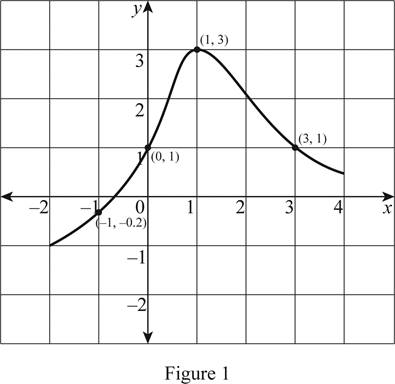# The value of f ( 1 ) .### Single Variable Calculus: Concepts...

4th Edition
James Stewart
Publisher: Cengage Learning
ISBN: 9781337687805### Single Variable Calculus: Concepts...

4th Edition
James Stewart
Publisher: Cengage Learning
ISBN: 9781337687805

#### Solutions

Chapter 1.1, Problem 1E

(a)

To determine

## The value of f(1).

Expert Solution

The value of f(1) is 3.

### Explanation of Solution

Given:

Plot the points in the given graph as shown below in Figure 1.Calculation:

In Figure.1, x-axis represents the values of x, and y-axis represents the values of f(x).

From Figure 1, it is noticeable that the point (1, 3) is plotted.

Therefore, the value of f(1)=3.

(b)

To determine

### The value of f(−1).

Expert Solution

The value of f(1) is approximately −0.2.

### Explanation of Solution

From Figure 1, it is seen that when x = −1 the f(x) is approximately equal to −0.2.

Therefore, the value of f(1)0.2.

(c)

To determine

### The value of x if f(x)=1.

Expert Solution

If f(x)=1, then the values of x are 0 and 3.

### Explanation of Solution

From Figure 1, it is it is noticeable that the points (0, 1) and (3, 1) are plotted.

Therefore, when f(x)=1 the values of x=0,3.

(d)

To determine

### The value of x if f(x)=1.

Expert Solution

If f(x)=1, then the values of x is approximately −0.8.

### Explanation of Solution

It is seen from the Figure 1 that when f(x) = 0, the value of x is approximately −0.8.

Therefore, when f(x)=1 the value of x0.8.

(e)

To determine

### The domain and range of f.

Expert Solution

The domain of f is [2,4] and range of f is [1,3].

### Explanation of Solution

Since, the domain of a function is the set of all x values of the graph, the domain is [2,4]. In the interval notation, it can be written as 2x4.

Since, the range of a function is the set of all y values of the graph, the range of the function is [1,3]. In the interval notation, it can be written as −1 ≤ y ≤ 3.

(f)

To determine

Expert Solution